# Lesson eee: The Dominant Seventh Chord Introduction

 Lesson EEE: The Dominant Seventh Chord Introduction: The dominant seventh (V7) is one of the most distinctive chords in the tonal repertoire. Because of its unique construction among diatonic seventh chords, it immediately defines and implies a key. On top of this, it creates a strong need for resolution to the tonic. In fact, the relationship between tonic and dominant is so engrained in our ears that composers are able to avoid, delay, and alter the moment of resolution to heighten or soften the level of tension in a piece. In this lesson, you will learn how to construct a dominant seventh chord in any key and in any inversion. Following this, we will discuss the resolution of the various tendency tones of the dominant seventh chord and, in turn, the chord as a whole. Construction: The dominant seventh chord is constructed by adding the chordal seventh (4) to the dominant triad. The following example shows the C-major dominant seventh chord in an SATB setting: Example 2: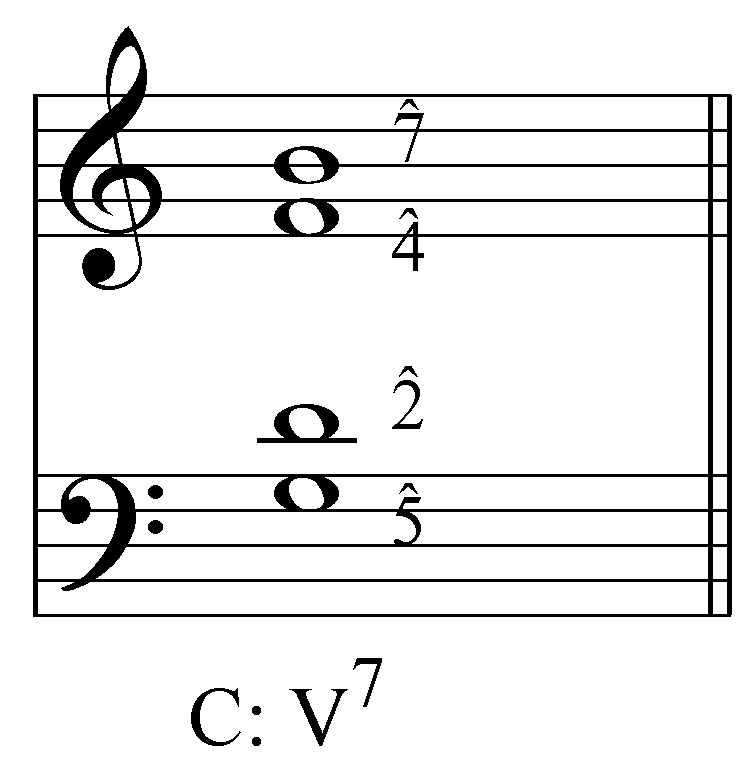The construction of the V7 is the same in minor. The following example shows a dominant seventh chord in A minor. Remember to raise the leading tone (G to G# in this case) for dominant chords in minor keys: Example 3: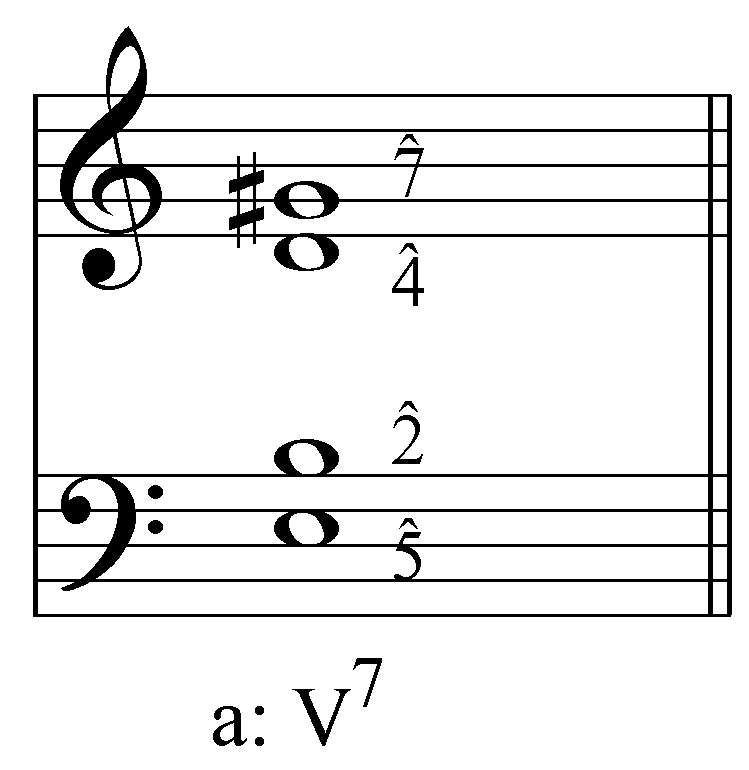[Potential activity: Have students construct or complete V7 chords in various keys.] Tendency tones: In lesson DDD we discussed the nature and function of tendency tones in music. As you’ll remember, tendency tones are those which require resolution due to their dissonant relation to the tones around them. There are two tendency tones in the V7 chord: scale degrees 7 and 4. These two tendency tones form a tritone—a dissonance that must be resolved properly and with care. Example 4: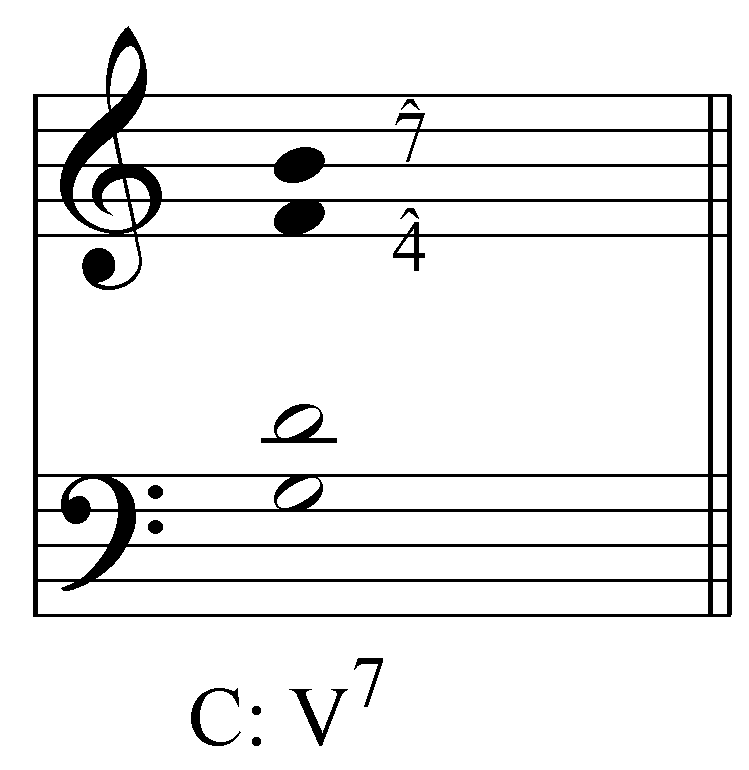[Potential activity: Give students a series of V7 chords in various keys/positions and have them identify the tendency tones. They might also be asked to identify the type of tritone formed.] Scale degree 7, the leading tone, has a particularly strong pull to scale degree 1. Except in one specific situation—discussed below—7 must resolve to 1. Scale degree four, the other tendency tone, by itself is not as strong as scale degree 7. It typically resolves downwards to 3. However, 4’s position as the chordal seventh also requires that it resolve downwards. These dual tendencies create a substantial need for resolution. The following example demonstrates the proper resolutions of tendency tones 4 and 7: Example 5: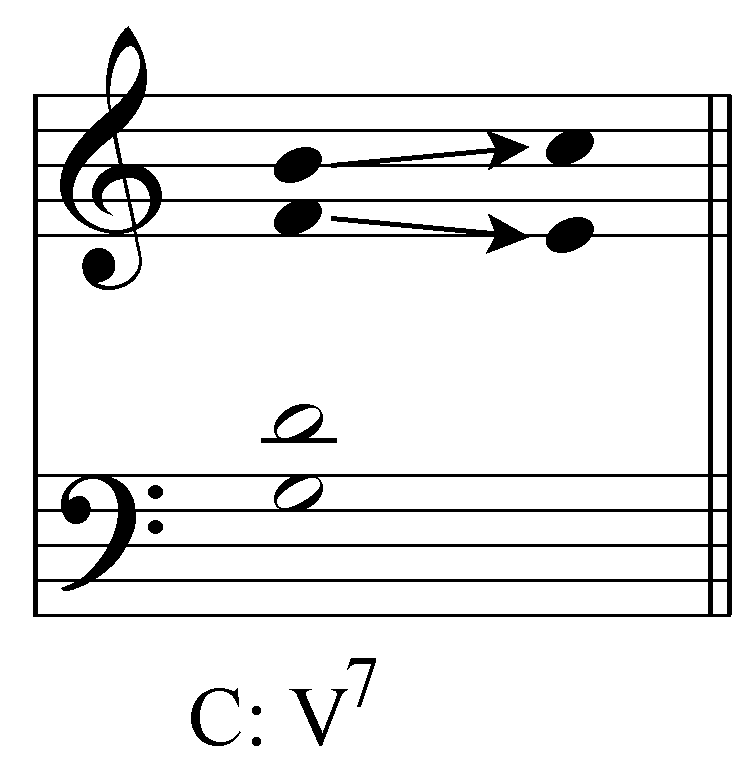In this case the augmented fourth formed by 4 and 7 resolve outwards to a sixth. (You may wish to refer back to lesson BBB where the tritone interval progressions are explored in greater depth. This particular example uses the progression from example 4 in lesson BBB.) [Potential activity: Have students resolve the identified tritones from the previous activity. Using the same examples will hopefully reinforce the continuity of the lesson.] There remain, however, two other notes in the V7 chord: the root (5) and the fifth (2). These two voices, forming a fifth in the V7 chord, usually resolve in similar motion to an octave. The following example shows the basic interval progressions in the upper and lower voice pairs: Example 6: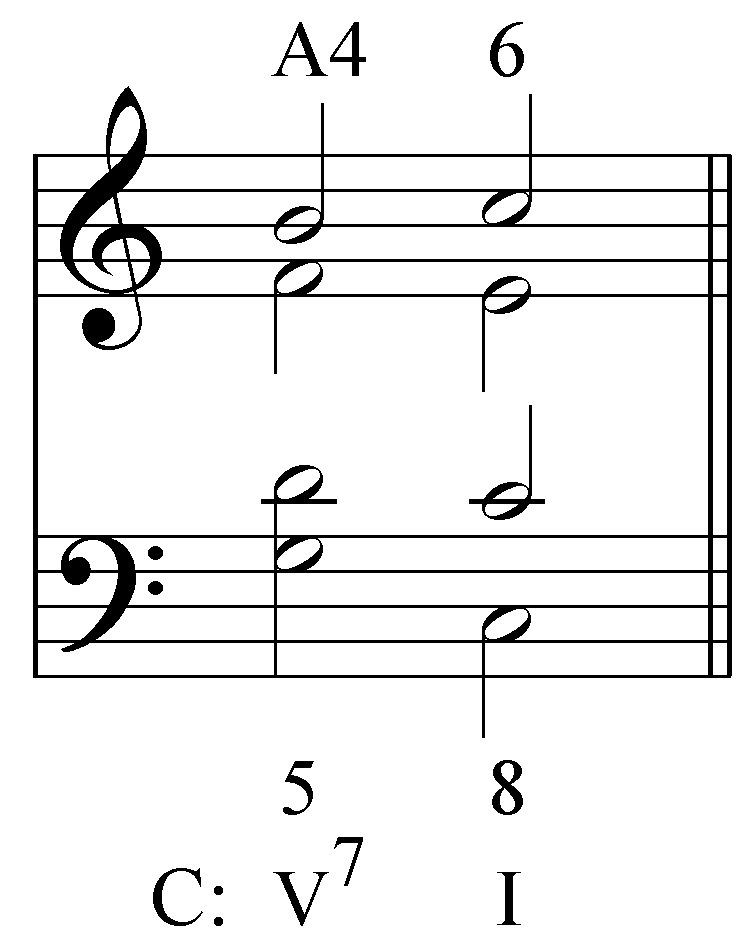It is helpful to simplify these patterns by looking at the motion in each voice: in a typical V7 - I cadence, 2 will resolve by step to 1 and 5 will leap down in the bass to 1. In the example above, you might have noticed that the chord of resolution has three roots, one third, and no fifth. This voicing of the I chord is a common and valid cadential idiom. This type of cadence, with both chords in root position, provides the greatest sense of repose. [Potential activity: Have students complete the I chord from each of the above exercises.] Inversions: The V7 chord is often found in inversion. While the preferred resolutions of the tendency tones should remain the same regardless of the position of V7, the resolution of 5 and 2 are varied. Consider the following example, where a dominant seventh chord in first inversion resolves to the tonic triad: Example 7: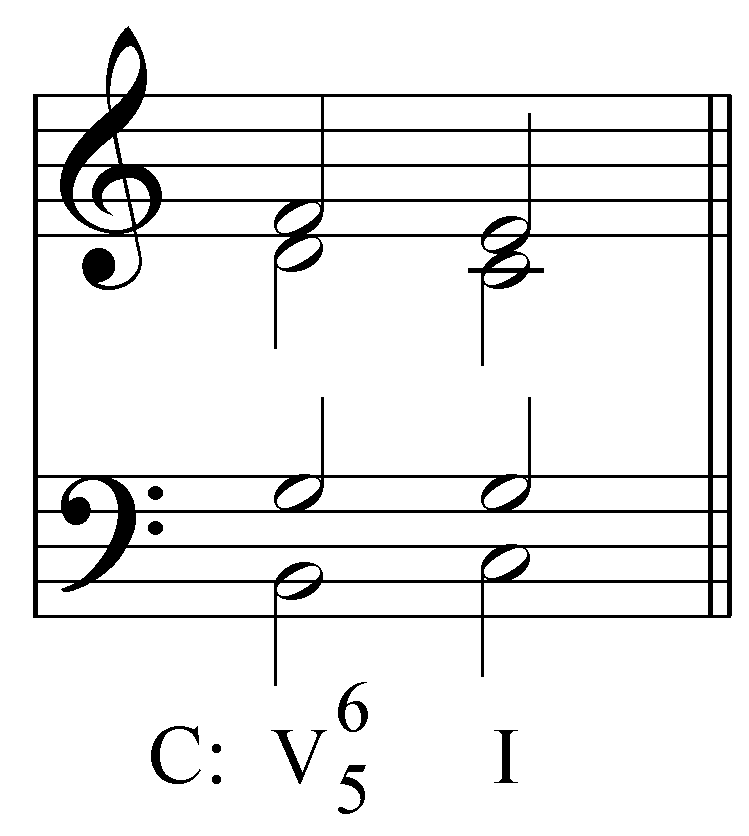In this example, both tendency tones resolve as expected: 7 to 1 in the bass and 4 to 3 in the soprano. As in example 6, 2 resolves to 1. In this case, however, because the root of the V7 chord (5) is not in the bass, we are free to sustain it as the fifth of the I chord. This is the preferred voice-leading since the held note lends a greater degree of smoothness to the resolution. The resolution of the dominant seventh in second inversion follows the same voice-leading patterns as example 7: 2 resolves to 1 in the bass, 5 is held in the tenor and the tendency tones are resolved in the alto and soprano: Example 8: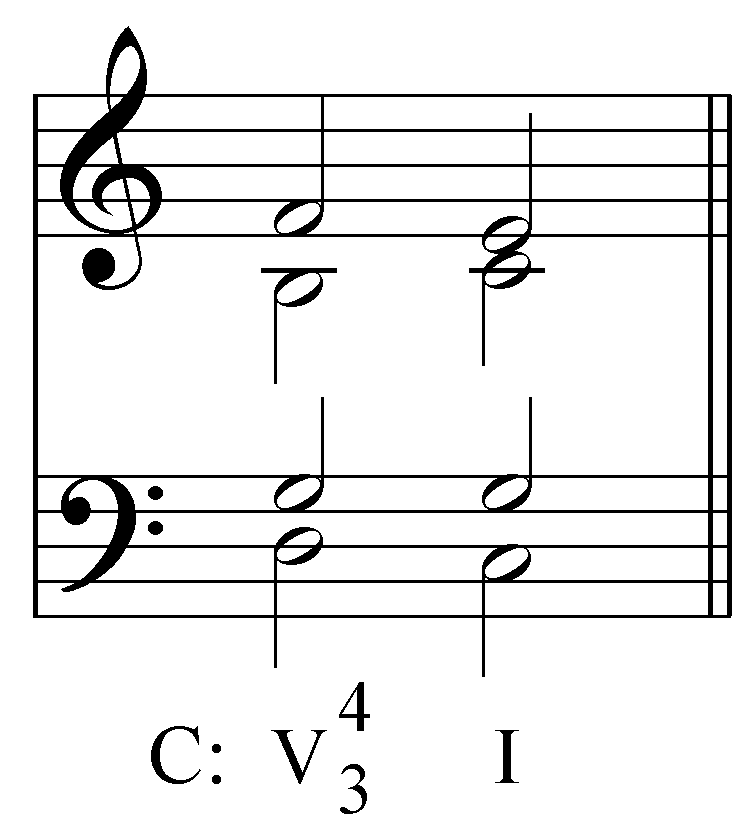Example 9 shows the resolution of the remaining position of the dominant seventh chord: Example 9: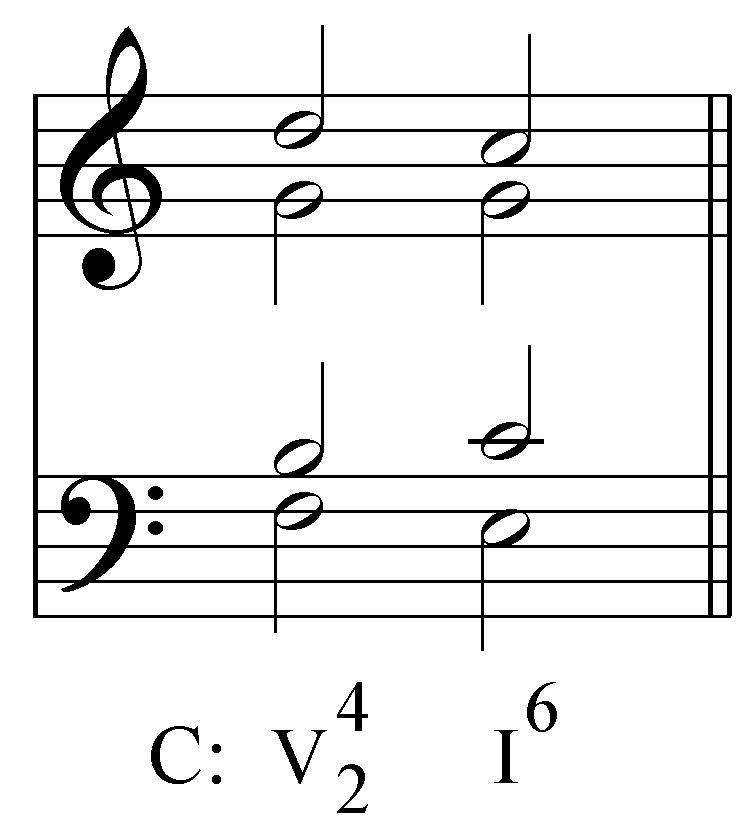In this case, because of tendency of 4 to resolve to 3, the chord of resolution is necessarily in first inversion. It should be noted that this formation of the V7 to I cadence is the least stable and requires further motion.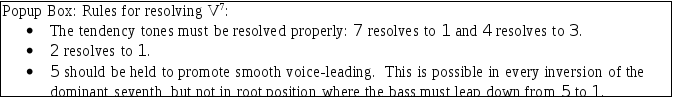[Potential activity: In a somewhat lengthier exercise than the above exercises, walk students through the resolution of a V65 chord. Following this, have students resolve a series of dominant seventh chords in various inversions (including root position) on their own.] Download 89.22 Kb.Share with your friends: